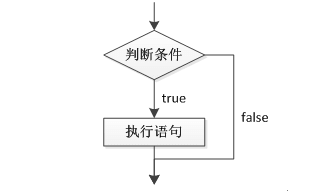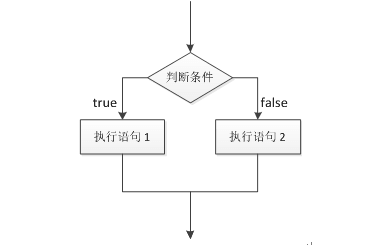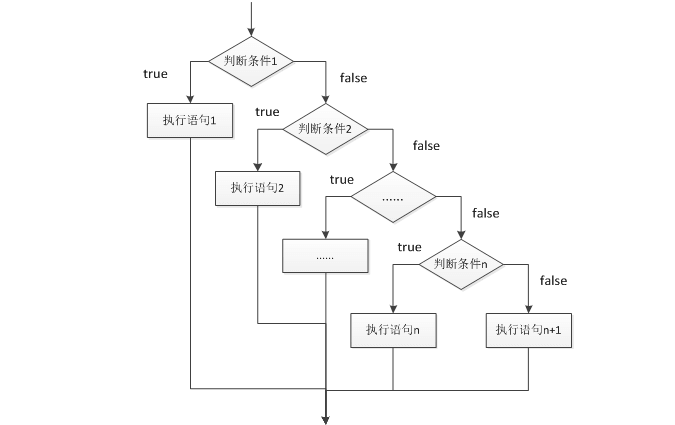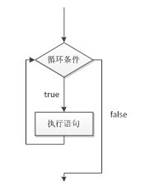JAVA基础二• 累计撰写 34 篇文章
• 累计收到 7 条评论

# JAVA基础二2021-06-21 / 0 评论 / 37 阅读 / 正在检测是否收录...

JAVA流程控制语句JAVA中非常重要的基础知识点务必完全理解掌握，总结记录一下之前学习笔记。JAVA流程控制语句是编程的基础知识，主要是为了更好的组织代码的执行流程，下面将详细介绍java流程控制语句。

### 一、选择结构if

if条件语句分为三种格式。

1. if语句if语句格式：

``````if（条件）{
语句的执行体
}``````

``````/**
* if语句例子
*/
public class IfDemo {

public static void main(String[] args) {
// TODO Auto-generated method stub
int i = 5;
if(i==5) {
System.out.println("if中的条件是true");
i++;
}
System.out.println("i="+i);
}

}``````
``````**注意：if()括号后面没有分号";"**
``````
1. if...else语句if ... else语法格式：

``````if(条件){
if的执行体
}else{
else的执行体
}``````

``````**
* if ... else 语句
*
*/
public class IfElseDemo {

public static void main(String[] args) {
int i = 13;
if(i%2==0) {
System.out.println(i+"是偶数");
}else {
System.out.println(i+"是奇数");
}
}

}``````
1. if...else if...else语句``````if(条件1){
执行语句1
}else if(条件2){
执行语句2
}
...
else if(条件n){
执行语句n
}else{
执行语句n+1
}``````

``````

``````    package java_learn_code;

import java.util.Scanner;

/**
* if...else if...else
* 多条件判断
*/
public class IfElseIfElseDemo {
public static void main(String[] args) {
while(true) {
System.out.print("请输入分数:");
Scanner scan = new Scanner(System.in);
double sum = scan.nextDouble();
if(sum<90) {
System.out.print("可以哦");
}else if(sum <=80) {
System.out.println("合格");
}else if(sum <=60){
System.out.println("不及格");
}else {
System.out.println("分数有问题");
}
System.out.println("");
}
}
}``````

### 二、三元运算符

``判断条件 ？ 表达式1 : 表达式2``

``````package java_learn_code;
/**
* if..else 与三元运算符的转换
*/
public class IfAllDemo {

public static void main(String[] args) {
int m = 10;
int n = 8;
//if...else运算
if(m>n) {
System.out.println(m);
}else {
System.out.println(n);
}

//三元运算符运算
System.out.println(m>n ? m : n);
}

}``````

### 三、循环语句while

while语句会反复地进行条件判断，只要条件成立，{}内的执行语句就会执行，直到条件不成立，while循环结束。while循环语句的语法结构:

``````while(循环条件){

………
}``````

``````/**
* while循环语句
*/
public class WhileDemo {

public static void main(String[] args) {
int i = 0;
while(i<5) {
System.out.println("i="+i);
i++;
}
}

}``````

### 四、循环语句for

``````for（初始化表达式; 循环条件; 操作表达式）{
执行语句
………
}``````

``````/**
* for循环
* @author Jole
*
*/
public class ForDemoOne {

public static void main(String[] args) {
//1、变量  2、条件 3、增量
for(int i=0;i<5;i++) {
System.out.println("i="+i); //两大括号之间：4、循环体
}
}

}``````

``````第一步：变量定义i，在整个循环中，只会执行一次（int i=0,在整个过程中至执行一次）。

``````for（1 ; 2 ; 3）{
4
}

``````1、初始化表达式、循环条件和操作表达式，它们之间用“;”分隔，{}中的执行语句为循环体。
2、int i=0；终其一生只运行一次。
3、条件为false时，不会在走增量i++，而是直接结束了。``````

``````/**
* 用for循环进行累加求和，1+2+3+4...+100=?
*/
public class ForSum {

public static void main(String[] args) {
int sum = 0;
for(int i=1;i<=100;i++) {
sum += i;
}
System.out.println("sum="+sum);
}
}``````

### 五、do...while()循环

do…while循环语句和while循环语句功能类似。

``````do {

………
} while(循环条件);``````

``````public class DowhileDemo {

public static void main(String[] args) {
int i = 1;
do {
i++;
}while(i<0);
System.out.println("i="+i);
}

}``````

do …while循环和while循环能实现同样的功能，区别在于，如果循环条件在循环语句开始时就不成立，那么while循环的循环体一次都不会执行，而do…while循环的循环体还是会执行一次。（无论条件是否成立，do...while都会执行一次循环体）

### 六、死循环(无限循环)

``````while(true){}

for(;;){}``````

``````/**
* while、for死循环
*/

public static void main(String[] args) {
//while死循环
while(true) {
}
//for死循环(注意：上面的while死循环，这个for死循环不会执行)
for( ;true ; ) {

}
}

}``````

### 七、循环嵌套

``````for(初始化表达式; 循环条件; 操作表达式) {
………
for(初始化表达式; 循环条件; 操作表达式) {
执行语句
………
}
………
}``````

``````public class ForForDemoTwo {

public static void main(String[] args) {
for(int i = 0;i < 2;i++) {
for(int j = 0; j<2 ;j++) {

}
}
}
}``````

``````1.i=0
2.i<2

3.执行循环体j=0
4.j<2
5.执行内循环的循环体
6.j++ 1

7.j<2
8.执行内循环的循环体
9.j++
10.j<2 内循环结束

11.外循环的增量i++ 1

12.i<2

13.外循环的增量i++ 2
14.i<2``````

``````public class ForForDemo {
public static void main(String[] args) {
for(int i=1;i<=5;i++) {
for(int j=1;j<i;j++) {
System.out.print("* ");
}
System.out.println("*");
}
}
}``````

*

• *
• *
• *
• *
``````

### 八、跳转语句(break、continue)

**跳转语句用于实现循环执行过程中程序流程的跳转，在Java中的跳转语句有break语句和continue语句。**

1.  **break语句**
``````

1、在switch条件语句和循环语句中都可以使用break语句。当它出现在switch条件语句中时，作用是终止某个case并跳出switch结构。
2、当它出现在循环语句中，作用是跳出循环语句，执行后面的代码。

public class BreakDemo {

``````   public static void main(String[] args) {
int i=1;
while(true) {
if(i==3) {
break;
}else {
System.out.println("i="+i);
}
i++;
}
}``````

}

``````   输出结果：
``````

i=1
i=2

``````

**多循环，break是结束的哪个循环?**
``````

public class MostForBreakDemo {

``````   public static void main(String[] args) {
for(int i=1;i<5;i++) {
for(int j=1;j<7;j++) {
if(j==4) {
break;
}else {
System.out.print(" j="+j);
}
}
System.out.println(" i="+i);
}
}``````

}

``````
**注意：当break语句出现在嵌套循环中的内层循环时，它只能跳出内层循环，如果想使用break语句跳出外层循环则需要对外层循环添加标记。

package java_learn_code;
public class MostForBreakDemo {

``````   public static void main(String[] args) {
a:for(int i=1;i<4;i++) {
for(int j=1;j<7;j++) {
if(j==4) {
break a;
}else {
System.out.print(" j="+j);
}
}
System.out.println(" i="+i);
}
}``````

}

``````   输出结果：
``````
``j=1 j=2 j=3``
``````
**switch或循环遇到break就全结束。**

2. **continue语句**

**continue语句用在循环语句中，它的作用是终止本次循环，执行下一次循环。**
``````

public class ContinueDemo {

``````   public static void main(String[] args) {
for (int j = 1; j < 7; j++) {
if (j == 4) {
continue;
} else {
System.out.print("  j=" + j);
}
}
}``````

}

``````
输出结果：
``````
`` j=1  j=2  j=3  j=5  j=6``
``````

``````

public class ContinueDemoTwo {

``````   public static void main(String[] args) {
a:for(int i=1;i<7;i++) {
for(int j=1;j<4;j++) {
if(i==3) {
continue a;
}else {
System.out.print("  j="+j);
}
}
System.out.println(" i="+i);
}
}``````

}

``````
输出结果：
``````
`````` j=1  j=2  j=3 i=1
j=1  j=2  j=3 i=2
j=1  j=2  j=3 i=4
j=1  j=2  j=3 i=5
j=1  j=2  j=3 i=6``````
``````
**注意：同样支持标记，跳过某一次循环。**

### 九、综合实例：随机猜数字``````

import java.util.Random;
import java.util.Scanner;

public class DemoOne {

``````   public static void main(String[] args) {
while(true) {
System.out.print("请输入猜的数字：");
Scanner scan = new Scanner(System.in);
int number = scan.nextInt();
Random ran = new Random();
int yourNum = ran.nextInt(10)+1;
if(number==yourNum) {
System.out.println("恭喜你猜对了，么么哒！");
break;
}else if(number>yourNum) {
System.out.println("输入的值："+number);
System.out.println("随机值："+yourNum);
System.out.println("sorry....猜大了哦");
}else {
System.out.println("输入值："+number);
System.out.println("随机值："+yourNum);
System.out.println("sorry....猜小洛");
}
System.out.println("");
}
}``````

}

``````### 十、选择结构switch

Switch语法格式:
``````

switch (表达式){

``````case 目标值1:
执行语句1
break;
case 目标值2:
执行语句2
break;
．．．．．．
case 目标值n:
执行语句n
break;
default:
执行语句n+1
break;``````

}

``````

switch语句将表达式的值与每个case中的目标值进行匹配，如果找到了匹配的值，会执行对应case后的语句，如果没找到任何匹配的值，就会执行default后的语句。break的作用是跳出switch语句。在switch语句中的表达式只能是byte、short、char、int类型的值，如果传入其它类型的值，程序会报错。

public class SwitchDemo {

``````public static void main(String[] args) {
boolean flag=true;
while(flag) {
System.out.print("星期转换：");
Scanner scan = new Scanner(System.in);
int num = scan.nextInt();
switch(num) {
case 1:
System.out.println("星期一");
break;
case 2:
System.out.println("星期二");
break;
case 3:
System.out.println("星期三");
break;
case 4:
System.out.println("星期四");
break;
case 5:
System.out.println("星期五");
break;
case 6:
System.out.println("星期六");
break;
case 7:
System.out.println("星期日");
break;
default:
flag = false;
System.out.println("退出成功！");
}
System.out.println("");
}
}``````

}

``````
Case的穿透性：在使用switch语句的过程中，如果多个case条件后面的执行语句是一样的，则该执行语句只需书写一次即可，这是一种简写的方式。

``````

import java.util.Scanner;
public class SwitchCaseDemo {

``````public static void main(String[] args) {
boolean flag=true;
while(flag) {
System.out.print("星期转换：");
Scanner scan = new Scanner(System.in);
int num = scan.nextInt();
switch(num) {
case 1:
case 2:
case 3:
case 4:
case 5:
System.out.println("工作日");
break;
case 6:
case 7:
System.out.println("休息日");
break;
default:
flag = false;
System.out.println("推出成功！");
}
System.out.println("");
}
}``````

}

1